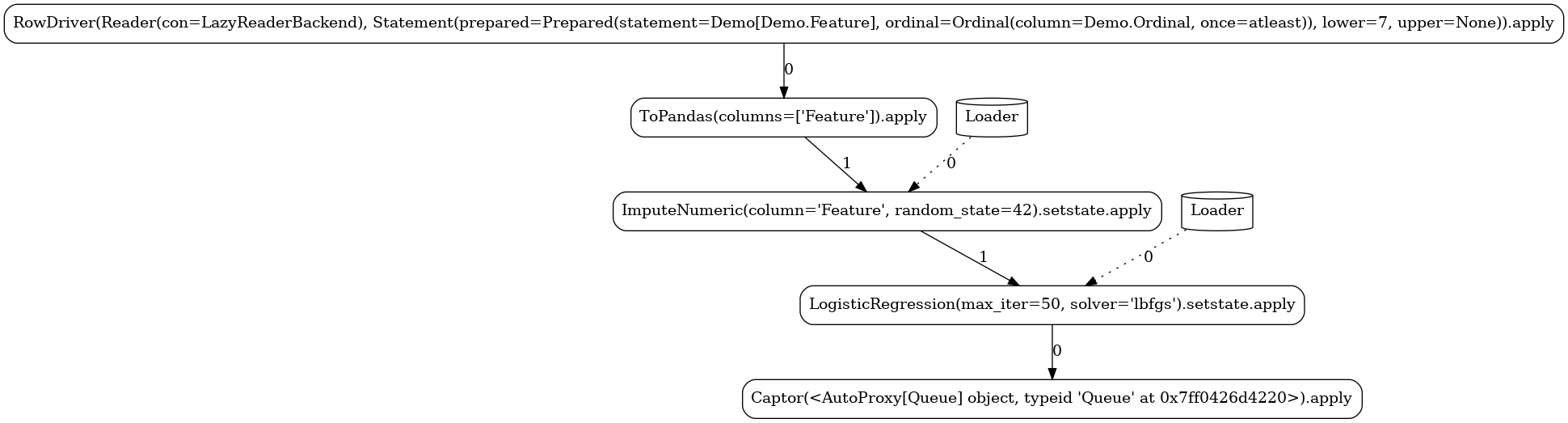# Pipeline Demos¶

This chapter presents a number of ForML pipelines demonstrating the workflow concept. These are stripped-down snippets focusing purely on the pipelining without constituting full-fledged ForML projects.

To visualize the composed workflow DAGs, we are going to execute the pipelines using the `Graphviz runner` via the interactive `runtime.Virtual` launcher.

Caution

Despite still being perfectly executable, all the operators as well as the actual dataset have been put together just to illustrate the topological principles without seeking true functional suitability in the first place.

Common Code

1. We define a dummy dataset schema with just three columns - a sequence ID of each record (`Ordinal`), the independent data point (`Feature`), and a hypothetical outcome (`Label`).

2. Using this dataset, we load it as inline data into the `Monolite feed` that we will be explicitly engaging when launching each of the demos.

3. Finally, we define the standard `Source descriptor` by specifying the features DSL query plus the label and ordinal columns.

Note

Only the last step (the `Source descriptor` declaration) would normally be part of project implementation. All the data provisioning (the feed setup) would be delivered independently via the configured platform.

tutorials/demos/__init__.py
 ``` 1 2 3 4 5 6 7 8 9 10 11 12 13 14 15 16 17 18 19 20 21 22 23 24``` ``````from forml import project from forml.io import dsl from forml.pipeline import payload from forml.provider.feed import monolite class Demo(dsl.Schema): """Demo dataset schema.""" Ordinal = dsl.Field(dsl.Integer()) Label = dsl.Field(dsl.Integer()) Feature = dsl.Field(dsl.Integer()) #: Demo dataset. DATA = [[3, 1, 10], [4, 0, 11], [5, 1, 12], [6, 0, 13], [7, 1, 14], [8, 0, 15]] #: Demo Feed preloaded with the DATA represented by the Demo schema FEED = monolite.Feed(inline={Demo: DATA}) #: Common Source component for all the demo pipelines SOURCE = project.Source.query(Demo.select(Demo.Feature), Demo.Label, ordinal=Demo.Ordinal) >> payload.ToPandas( columns=['Feature'] ) ``````

## Mini¶

Starting with a minimal use case, this mini-pipeline contains just a single (stateful) operator - the `RandomForestClassifier` imported from the SKLearn library under the operator auto-wrapping context which turns it transparently into a true ForML operator.

To launch the pipeline, it is first `bound` to the ```Source descriptor``` (declared previously in the common section) to dynamically assemble a virtual `Artifact` that directly exposes a `launcher instance`. We select the `Graphviz runner` (called `visual` in our setup) and explicitly provide our shared Demo feed (since that is not a platform-wide configured feed).

Below, you can see the different task graphs produced for each of the train versus apply modes (note the ordinal lower/upper bounds specified when executing each of the modes used as data filters with respect to the `Data.Ordinal` column). Apart from the expected `RandomForestClassifier` node, the topology contains a number of additional tasks:

tutorials/demos/mini.py
 ``` 1 2 3 4 5 6 7 8 9 10``` ``````import demos from forml.pipeline import wrap with wrap.importer(): from sklearn.ensemble import RandomForestClassifier PIPELINE = RandomForestClassifier(max_depth=3) LAUNCHER = demos.SOURCE.bind(PIPELINE).launcher('visual', feeds=[demos.FEED]) ``````
``````LAUNCHER.train(3, 6)
``````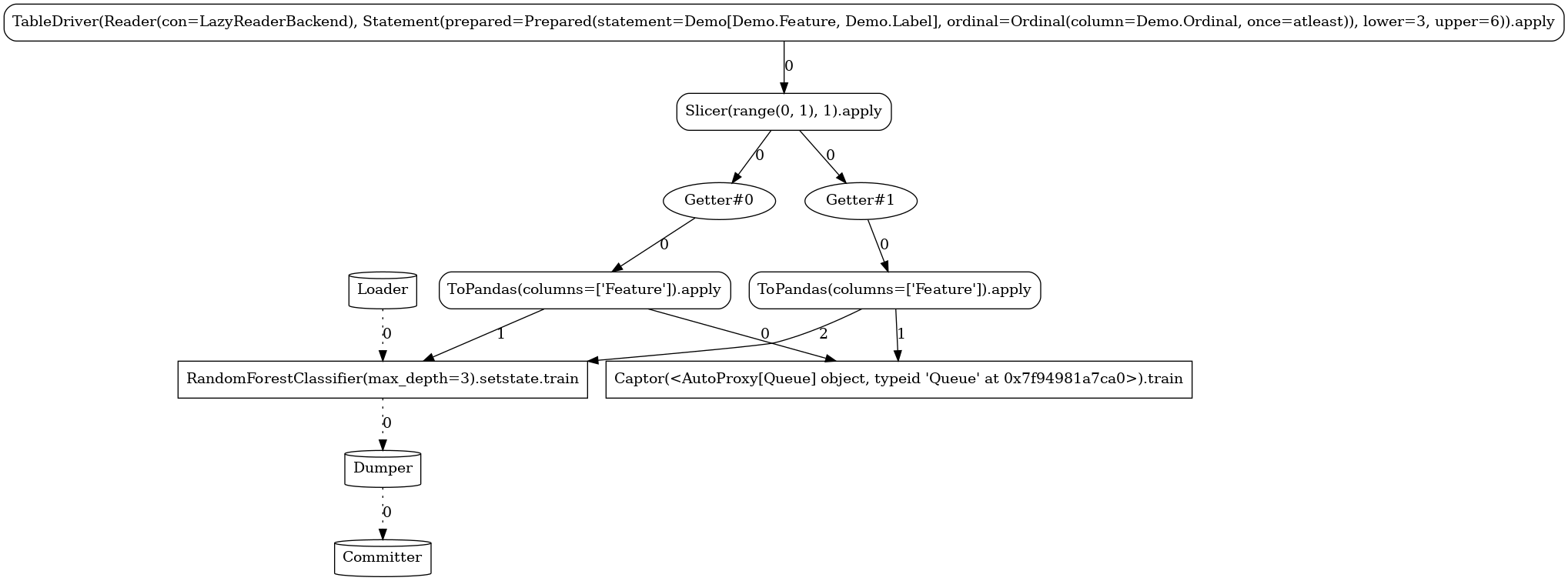``````LAUNCHER.apply(7)
``````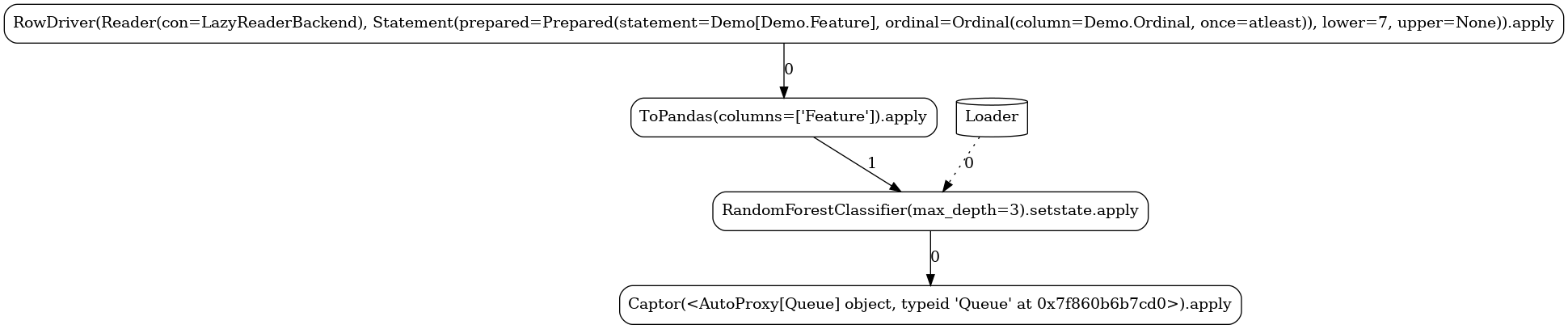## Simple¶

The next pipeline is demonstrating a true workflow expression of two (again stateful) operators participating in an operator composition:

tutorials/demos/simple.py
 ``` 1 2 3 4 5 6 7 8 9 10 11``` ``````import demos from forml.pipeline import wrap with wrap.importer(): from sklearn.impute import SimpleImputer from sklearn.linear_model import LogisticRegression PIPELINE = SimpleImputer(strategy='mean') >> LogisticRegression(max_iter=50, solver='lbfgs') LAUNCHER = demos.SOURCE.bind(PIPELINE).launcher('visual', feeds=[demos.FEED]) ``````
``````LAUNCHER.train(3, 6)
``````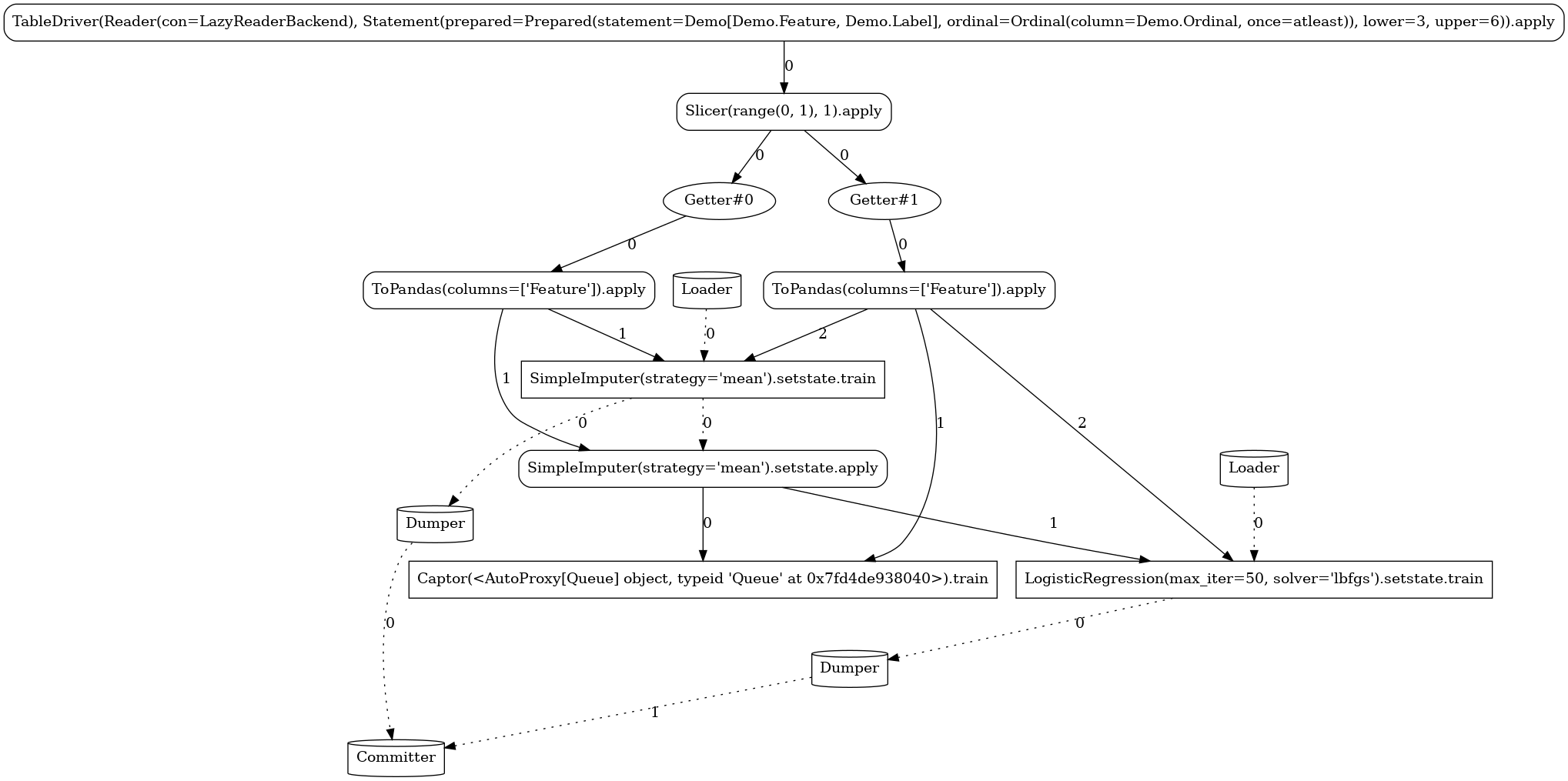``````LAUNCHER.apply(7)
``````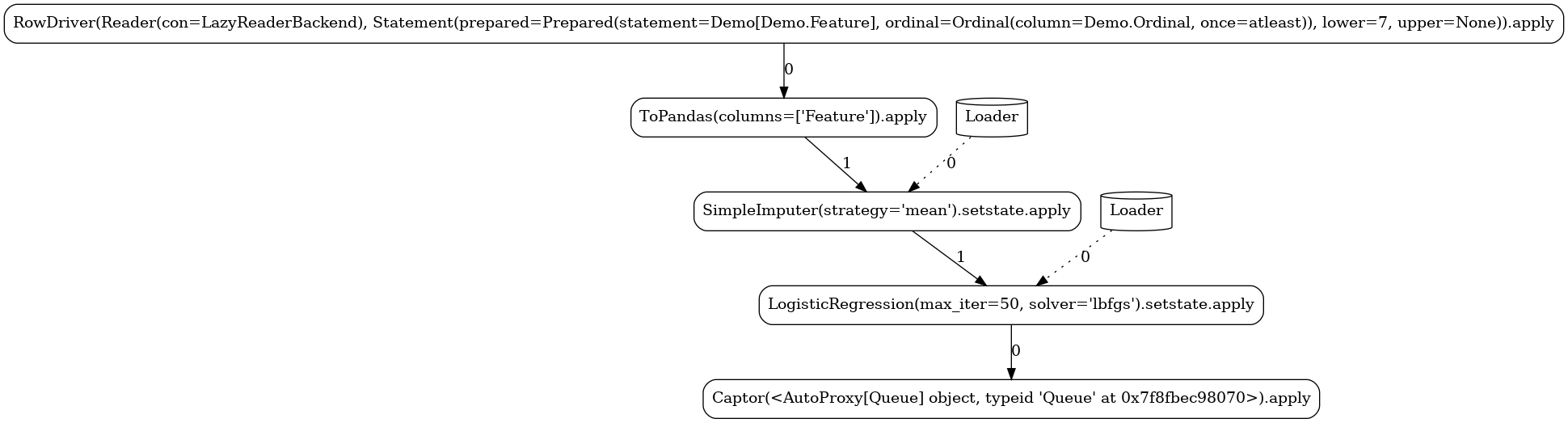## Ensemble¶

The ability to derive fairly involved workflows from simple expressions is demonstrated in the following pipeline employing the `model ensembling` technique. For readability, we use just two levels of folding (`n_splits=2`), the graph would be even more complex with higher folding levels.

tutorials/demos/ensemble.py
 ``` 1 2 3 4 5 6 7 8 9 10 11 12 13 14 15 16 17 18 19 20``` ``````# pylint: disable=ungrouped-imports import demos from sklearn import model_selection from forml.pipeline import ensemble, wrap with wrap.importer(): from sklearn.ensemble import GradientBoostingClassifier, RandomForestClassifier from sklearn.impute import SimpleImputer from sklearn.linear_model import LogisticRegression STACK = ensemble.FullStack( RandomForestClassifier(max_depth=3), GradientBoostingClassifier(max_depth=3), crossvalidator=model_selection.StratifiedKFold(n_splits=2), ) PIPELINE = SimpleImputer(strategy='mean') >> STACK >> LogisticRegression(max_iter=50, solver='lbfgs') LAUNCHER = demos.SOURCE.bind(PIPELINE).launcher('visual', feeds=[demos.FEED]) ``````
``````LAUNCHER.train(3, 6)
````````````LAUNCHER.apply(7)
``````## Complex¶

Going one step further, the following pipeline is again using the model ensembling technique, but this time it defines a distinct transformation chain for each of the model branches (`OneHotEncoder` for the `RandomForestClassifier` and `Binarizer` for `BernoulliNB`).

tutorials/demos/complex.py
 ``` 1 2 3 4 5 6 7 8 9 10 11 12 13 14 15 16 17 18 19 20 21 22``` ``````# pylint: disable=ungrouped-imports import demos from sklearn import model_selection from forml.pipeline import ensemble, wrap with wrap.importer(): from sklearn.ensemble import RandomForestClassifier from sklearn.impute import SimpleImputer from sklearn.linear_model import LogisticRegression from sklearn.naive_bayes import BernoulliNB from sklearn.preprocessing import Binarizer, OneHotEncoder FH_RFC = OneHotEncoder(handle_unknown='ignore') >> RandomForestClassifier(n_estimators=20, n_jobs=4, max_depth=3) BIN_BAYES = Binarizer(threshold=0.63) >> BernoulliNB(alpha=1.1) STACK = ensemble.FullStack(FH_RFC, BIN_BAYES, crossvalidator=model_selection.StratifiedKFold(n_splits=2)) PIPELINE = SimpleImputer(strategy='mean') >> STACK >> LogisticRegression(max_iter=50, solver='lbfgs') LAUNCHER = demos.SOURCE.bind(PIPELINE).launcher('visual', feeds=[demos.FEED]) ``````
``````LAUNCHER.train(3, 6)
``````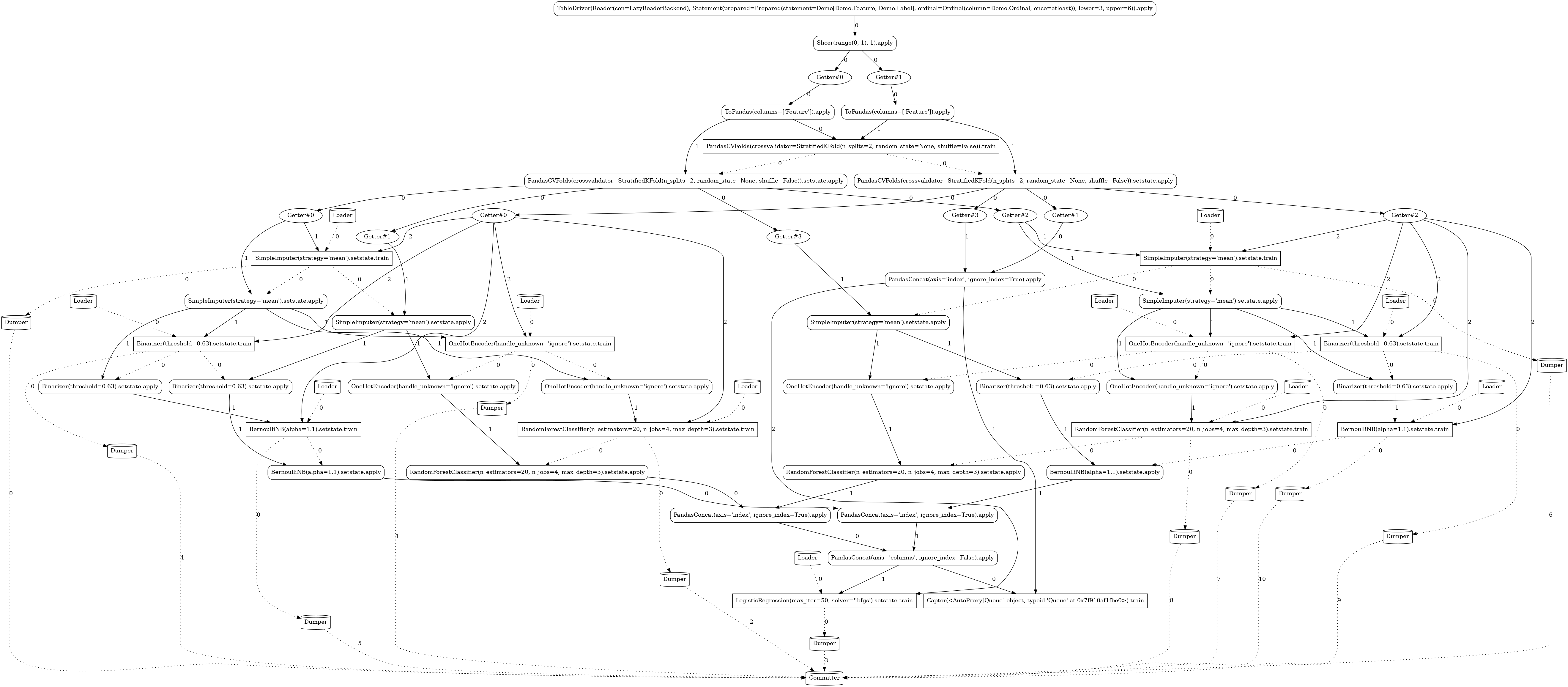``````LAUNCHER.apply(7)
``````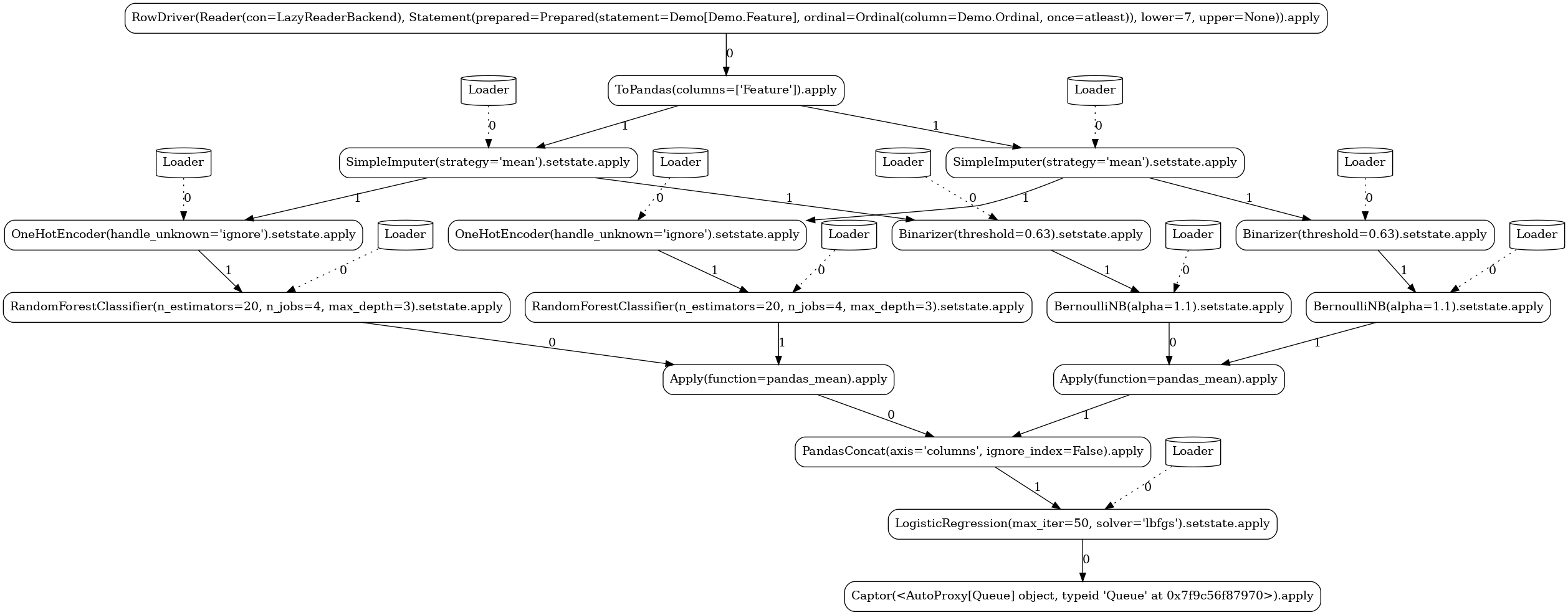## Custom¶

In this pipeline, we demonstrate the ability to define custom actors and operators. We implement a simple stateful mapper that at train mode persists the mean and standard deviation of the observed column and at apply mode generates random integers within the given `mean±std` range for any missing value.

tutorials/demos/custom.py
 ``` 1 2 3 4 5 6 7 8 9 10 11 12 13 14 15 16 17 18 19 20 21 22 23 24 25 26 27 28 29 30 31 32 33 34 35 36 37 38 39 40 41 42``` ``````import typing import demos import numpy as np import pandas as pd from forml.pipeline import wrap with wrap.importer(): from sklearn.linear_model import LogisticRegression @wrap.Actor.train def ImputeNumeric( state: typing.Optional[dict[str, typing.Any]], X: pd.DataFrame, y: pd.Series, column: str, random_state: typing.Optional[int] = None, ) -> dict[str, typing.Any]: """Train part of a stateful transformer for missing numeric column values imputation.""" return {'mean': X[column].mean(), 'std': X[column].std()} @wrap.Operator.mapper @ImputeNumeric.apply def ImputeNumeric( state: dict[str, typing.Any], X: pd.DataFrame, column: str, random_state: typing.Optional[int] = None ) -> pd.DataFrame: """Apply part of a stateful transformer for missing numeric column values imputation.""" na_slice = X[column].isna() if na_slice.any(): rand_age = np.random.default_rng(random_state).integers( state['mean'] - state['std'], state['mean'] + state['std'], size=na_slice.sum() ) X.loc[na_slice, column] = rand_age return X PIPELINE = ImputeNumeric(column='Feature', random_state=42) >> LogisticRegression(max_iter=50, solver='lbfgs') LAUNCHER = demos.SOURCE.bind(PIPELINE).launcher('visual', feeds=[demos.FEED]) ``````
``````LAUNCHER.train(3, 6)
````````````LAUNCHER.apply(7)
``````